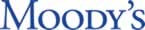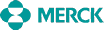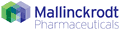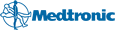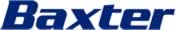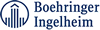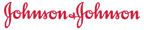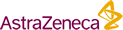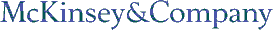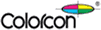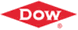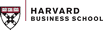Last Updated: January 26, 2022

DrugPatentWatch Database Preview

Claims for Patent: 4,889,919

« Back to Dashboard

Summary for Patent: 4,889,919
 Title: Biologically active PDGF derived A-chain homodimers Abstract: Dimeric proteins having substantially the same biological activity as PDGF are disclosed. More specifically, the protein may have two substantially identical polypeptide chains, each of the chains being substantially homologous to the A-chain of PDGF. Alternatively, the protein may have two polypeptide chains that are substantially identical to the A-chain of PDGF. In addition, proteins comprising polypeptides that are variants or derivatives of the A-chain of PDGF are also disclosed. Therapeutic compositions containing these proteins and methods for enhancing the wound-healing process in warm-blooded animals are also disclosed. Inventor(s): Murray; Mark J. (Seattle, WA), Kelly; James D. (Seattle, WA) Assignee: ZymoGenetics, Inc. (Seattle, WA) Application Number: 06/942,484 Patent Claims: 1. A substantially pure protein having two disulfide-bonded polypeptide chains, each of said chains comprising the amino acid sequence: V X1 K T R T V I Y E I P R S Q V D P T S A N F L I W F P X2 V E V K R X3 T G X4 X5 X6 T S S V K C Q P S R V H H R S V K V A K V E Y V R K K P K L K E V Q V R L E E H L E C A C A T T S L N P D Y R E wherein each of X1, X2, X3, X4, X5, and X6 is any amino acid. 2. A substantially pure protein having two disulfide-bonded polypeptide chains, each of said chains comprising the amino acid sequence: Q V D P T S A N F L I W P P X2 V E V K R X3 T G X4 X5 X6 T S S V K C Q P S R V H H R S V K V A K V E Y V R K K P K L K E V Q V R L E E H L E C A C A T T S L N P D Y E wherein each of X2, X3, X4, X5, and X6 is any amino acid. 3. A substantially pure protein having two disulfide-bonded polypeptide chains, each of said chains comprising the amino acid sequence: V X1 K T R T V I Y E I P R S Q V D P T S A N F L I W P P X2 V E V K R X3 T G X4 X5 X6 T S S V K C Q P S R V H H R S V K V A K V E Y V R K K P K L K E V Q V R L E E H L E C A C A T wherein each of X1, X2, X3, X4, X5, and X6 is any amino acid. 4. A substantially pure protein having two disulfide-bonded polypeptide chains, each of said chains comprising the amino sequence: Q V D P T S A N F L I W P P X2 V E V K R X3 T G X4 X5 X6 T S S V K C Q P S R V H H R S V K V A K V E Y V R K K P K L K E V Q V R L E E H L E C A C A T wherein each of X2, X3, X4, X5 and X6 is any amino acid. 5. A substantially pure protein having two disulfide-bonded polypeptide chains, each of said chains comprising the amino acid sequence. S I E E A V P A V X1 K T R T V I Y E I P R S Q V D P T S A N F L I W P P X2 V E V K R X3 T G X4 X5 X6 T S S V K C Q P S R V H H R S V K V A K V E Y V R K K P K L K E V Q V R L E E H L E C A C A T wherein each of X1, X2, X3, X4, X5 and X6 is any amino acid. 6. A substantially pure protein having two disulfide-bonded polypeptide chains, each of said chains comprising the amino acid sequence: S I E E A V P A V X1 K T R T V I Y E I P R S Q V D P T S A N F L I W P P X V E V K R X3 T G X4 X5 X6 T S S V K C Q P S V H H S V V A K V E Y V R K K P K L K E Q V R L E E H L E C A C A T T S L N P D Y R E wherein each of X1, X2, X3, X4, X5 and X6 is any amino acid. 7. A protein according to any one of claims 1 to 48 wherein said protein is unglycosylated. 8. A protein according to any one of claims 1 to 48 wherein X6 is a glutamine residue. 9. A protein according to any one of claims 3, 5 and 6 wherein X1 is a serine residue. 10. A composition comprising a protein according to any of claims 1-9 and a physiologically acceptable carrier or diluent. 11. The composition of claim 10 wherein said carrier or diluent is selected from the group consisting of albumin, sterile water and saline. 12. The composition of claim 10, further comprising an adjuvant. 13. The composition of claim 12 wherein said adjuvant is selected from the group consisting of collagen, hyaluronic acid, fibronection, factor XIII, and an antibiotic. 14. The composition of claim 10 wherein said protein is present in a concentration of from about 10 ng/ml to about 100 ug/ml of total volume.

Details for Patent 4,889,919

Applicant Tradename Biologic Ingredient Dosage Form BLA Approval Date Patent No. Expiredate
Merck Sharp & Dohme Corp. ZOSTAVAX zoster vaccine live For Injection 125123 2006-05-25 2004-10-12
>Applicant >Tradename >Biologic Ingredient >Dosage Form >BLA >Approval Date >Patent No. >Expiredate

Make Better Decisions: Try a trial or see plans & pricing